# Mass-spring system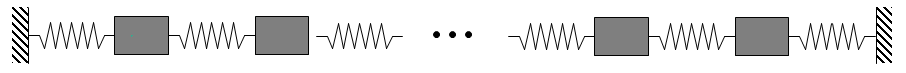Mass-spring system with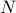masses on a line.

For the system withmasses on a line, the state vector is determined by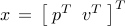, where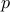and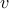denote the vectors of positions and velocities of all masses. Setting all masses and spring constants to unity and partitioning matrices in the state-space representation conformably with the partition of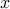yields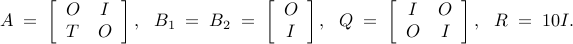where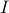and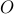are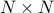identity and zero matrices, and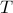is a tridiagonal symmetric Toeplitz matrix with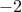on the main diagonal andon the first upper and lower sub-diagonals, e.g.,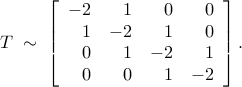In the absence of sparsity constraints, i.e., at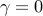, the optimal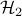controller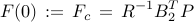is obtained from the positive definite solution of the algebraic Riccati equation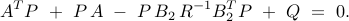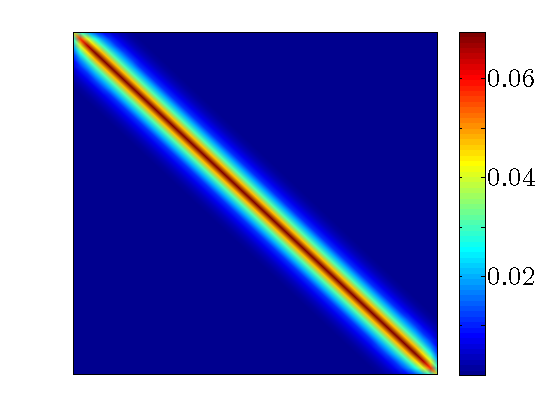The optimal centralized position feedback gain matrix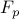in the mass-spring system with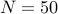masses. Bothand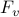in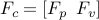have almost constant diagonals (modulo edges) and exponential off-diagonal decay.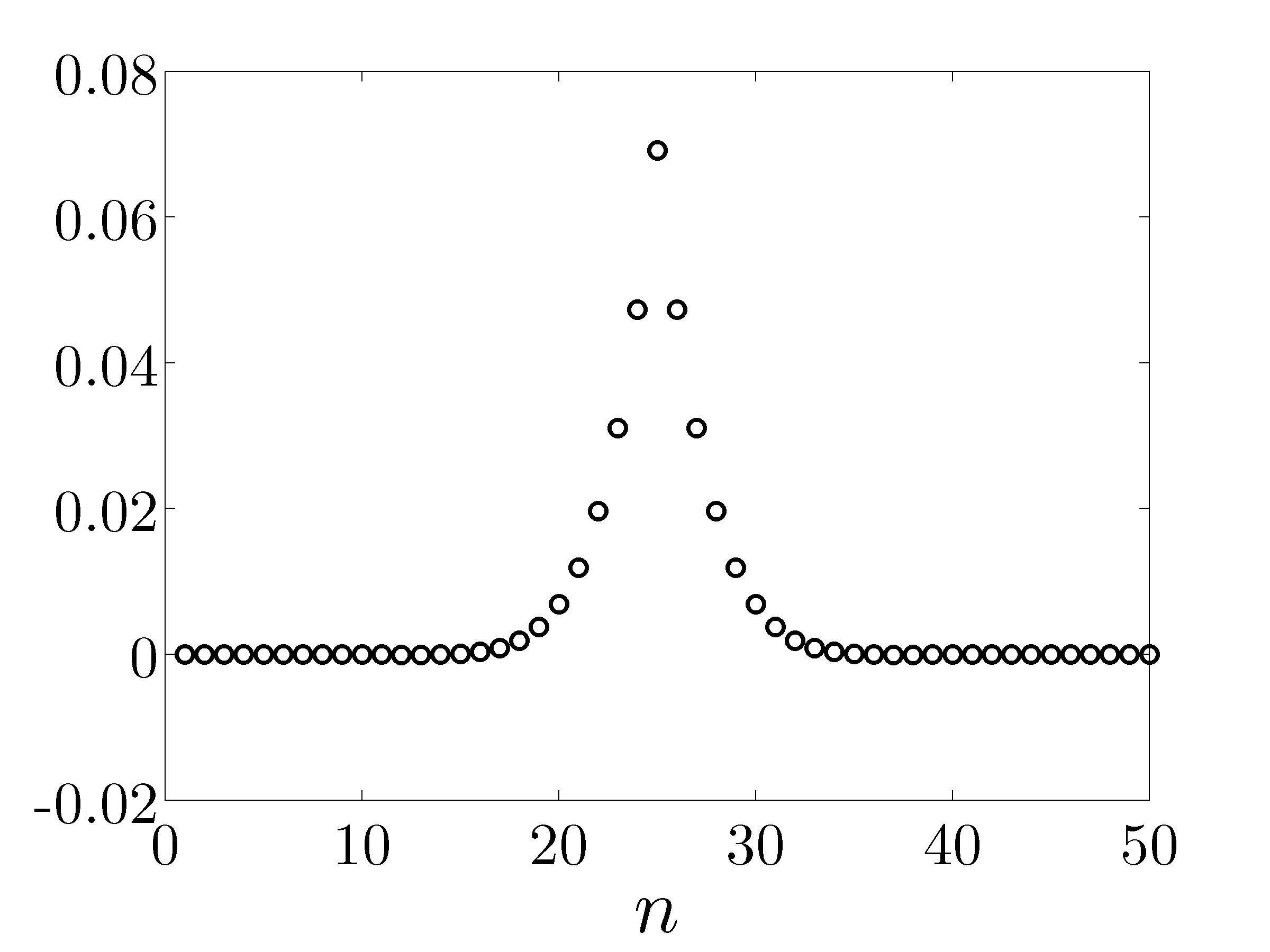Optimal centralized position gains for the middle mass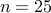. Note exponential decay with the spatial distance.

Localization can be enforced by truncating the optimal centralized controller. This, however, may introduce performance degradation and even instability of the closed-loop system. (Additional information is provided in the Network with 100 unstable nodes example.) Instead of using simple truncation, the alternating direction method of multipliers enforces sparsity in the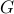-minimization step and improves the quadratic performance in the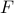-minimization step. This mechanism of alternating between promoting sparsity and optimizing the quadratic performance plays an important role in striking a balance between these two competing objectives.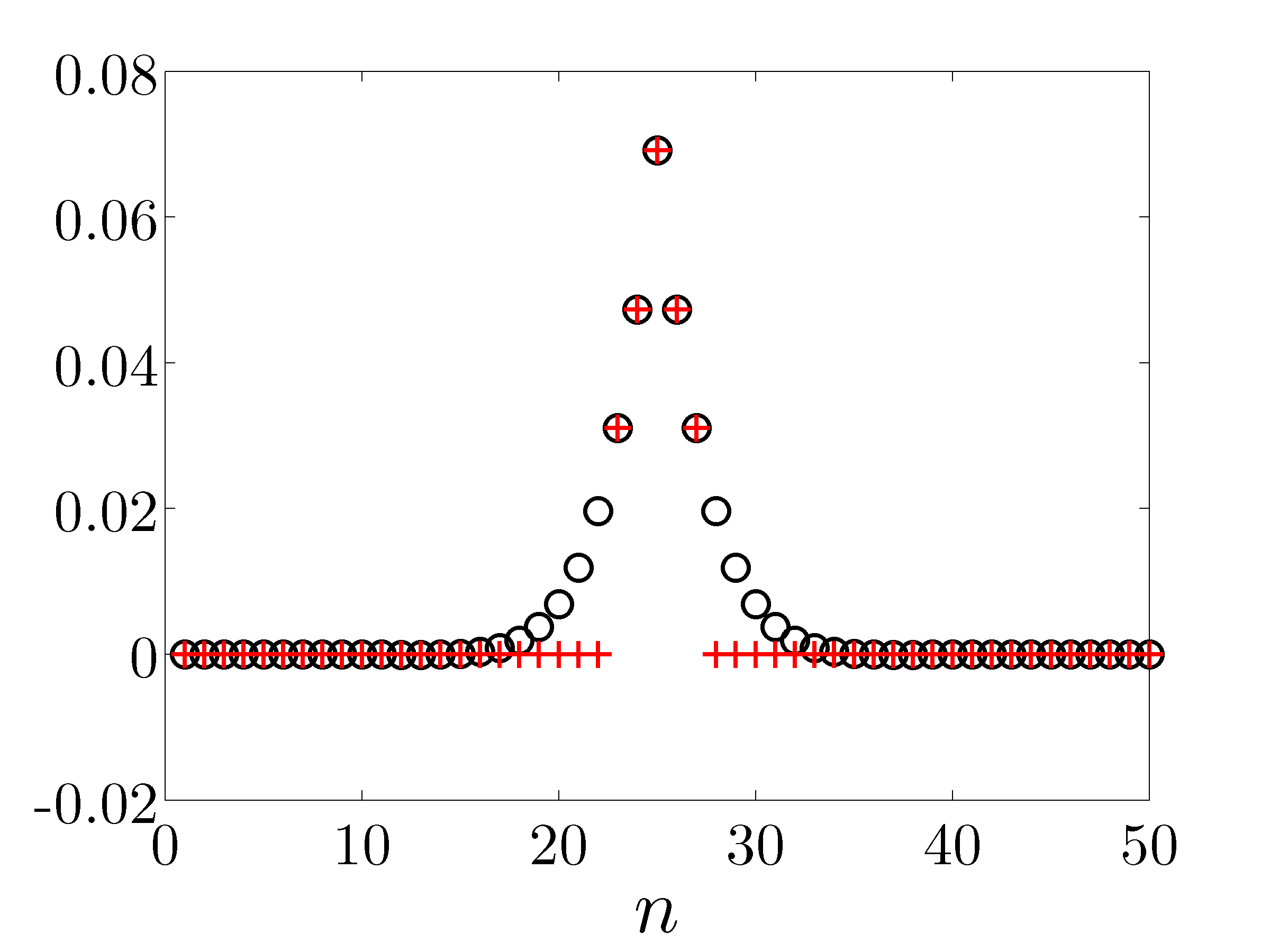An example of truncation: all but five largest elements of the optimal centralized position gain are set to zero. The resulting controller will only use the position measurements of the mass on which it acts and of the two neighboring masses to the left and to the right.

For illustration, we use cardinality function, weighted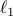norm, and sum-of-logs as the sparsity-promoting penalty functions. For all three cases, the optimal position and velocity feedback gains become diagonal matrices for large values of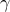. It is noteworthy that the optimal sparse feedback gain, with only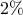of nonzero elements relative to the optimal centralized controller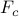, introduces performance loss of only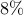(compared to).

The following links contain Matlab codes and computational results for each case.